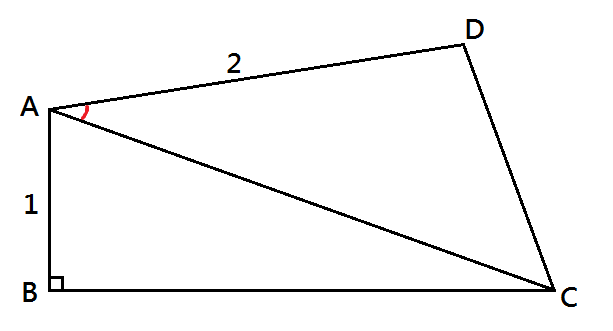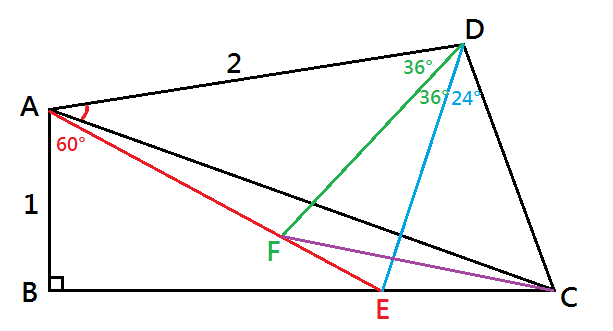## 求角度 (二)數學謎題30°

#### 解析首先，算得 ∠DCE = 78°。作第一次輔助線：在 BC 上畫一點 E 使 AE = 2 ，連 AE再連 DE。這樣一來 △ABE 是 邊長比 1 : √3 : 2 的直角三角形，故 ∠AEB = 30°，∠BAE = 60°，∠DAE = 36°。
• 由上可得 ∠DAE = 36°。又因 AD = AE = 2 ，得 △ ADE 是等腰三角形；故 ∠ADE = ∠ AED = 72°。
• 由上得到 ∠DEC = 78° ，得 △ DEC 是等腰三角形；故 DE = DC ，且 ∠ CDE = 24°。

• 由上得到 ∠ADF = 36°，得 △ ADF 是等腰三角形；故 AF = DF ，且 ∠ CDE = 24°。
• 另一方面，△ DEF 也是等腰三角形 (剛好與 △ADE 相似，都是黃金比例三角形)；故 DF = DE，∠DFE = 72°。
• 因此由上得到 DF = DC；加上有 ∠CDF = 60°，得 △DCF 是正三角形；故 CF = DF， ∠ DFC = 60°，∠CFE = 12 °。
• 由上得到 CF = AF，故△ AFC 是等腰三角形；故 ∠FAC = ∠ FCA 。
• 但是因為 ∠FAC + ∠ FCA = ∠CFE = 12 °，故由上可得 ∠FAC = 6° ，因此 ∠ DAC = 30°。

5,120